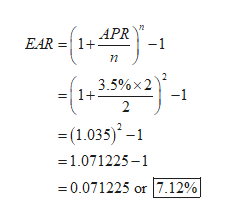# Your company has decided to set up a fund for its employees with an initial payment of Rs 27,500 compounded six-monthly over a four-year period at a six monthly interest of 3.5%.(a) Calculate the size of the fund at the end of 4 years.(b) Calculate the effective annual interest rate.iv) Show that P = A/r. for an ordinary annuity to infinity.

Question
6 views

Your company has decided to set up a fund for its employees with an initial payment of Rs 27,500 compounded six-monthly over a four-year period at a six monthly interest of 3.5%.
(a) Calculate the size of the fund at the end of 4 years.
(b) Calculate the effective annual interest rate.
iv) Show that P = A/r. for an ordinary annuity to infinity.

check_circle

Step 1

a.

Calculate the size of the fund as follows:

Step 2

b.

Calculate the effective annu...help_outlineImage TranscriptioncloseAPR 1+ EAR -1 2 3.5%x2 1+ -1 2 =(1.035) -1.071225-1 =0.071225 or 7.12%| fullscreen

### Want to see the full answer?

See Solution

#### Want to see this answer and more?

Solutions are written by subject experts who are available 24/7. Questions are typically answered within 1 hour.*

See Solution
*Response times may vary by subject and question.
Tagged in